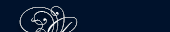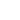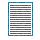НОВОСТИ   БИБЛИОТЕКА   ЭНЦИКЛОПЕДИЯ   КАРТА САЙТА   ССЫЛКИ   О САЙТЕ## FRICTION

Whenever a body slides along another body, a resisting force is called into play which is known as the force of friction.

This is a very important force and serves many useful purposes, for a person could not walk without it, or a car could not propel itself along the road without the friction between the tires and the road. On the other hand, friction is very wasteful because it reduces the efficiency of machines, since work must be done to overcome it, and this energy is wasted as heat. The purpose of this experiment is to study the laws of sliding friction and to determine the coefficient of friction between two surfaces.

Friction is the resisting force encountered when one tries to slide one surface over another; this force acts along the tangent to the surface in contact. The force necessary to overcome friction depends on the nature of the materials in contact, their roughness or smoothness, and on the normal force, but ^not on the area of contact, within wide limits. It is found experimentally that the force of friction is directly proportional to the normal force. The constant of proportionality is called the coefficient of friction.

The coefficient of friction is equal to the force of friction divided by the total normal force pressing the surfaces together. Thus

 К = F N

or F = KN where F is the force of friction to be overcome, N is the total normal force, or the perpendicular component of the force holding the two surfaces together, and К is the coefficient of friction.

To determine the above relation the substances must be in the form of a plane placed horizontally, with a pulley fastened at one end. The other substance is made in the form of a block to which a cord passing over the pulley and carrying weights is attached; these may be varied until the block moves uniformly when given a very slight push. The normal force between .the two surfaces can be changed by placing weights on top of the block, and the relation between the coefficient of friction, the force of friction, and the normal force can thus be tested.

The limiting angle of repose is the angle at which a body will just begin to slide down an inclined plane. The coefficient of friction is equal to the tangent of the angle of repose. It is found that the frictional force acting when actual sliding is taking place, is slightly lower than the maximum frictional force that can act just before the body begins to slide. Thus the kinetic coefficient of friction is somewhat lower than the static coefficient of friction.

in contact соприкасающиеся

is called into play зд. вступает в силу, появляется

necessary to overcome зд. необходимая, чтобы преодолеть

when given a very slight push если их слегка подтолкнутьПОИСК: